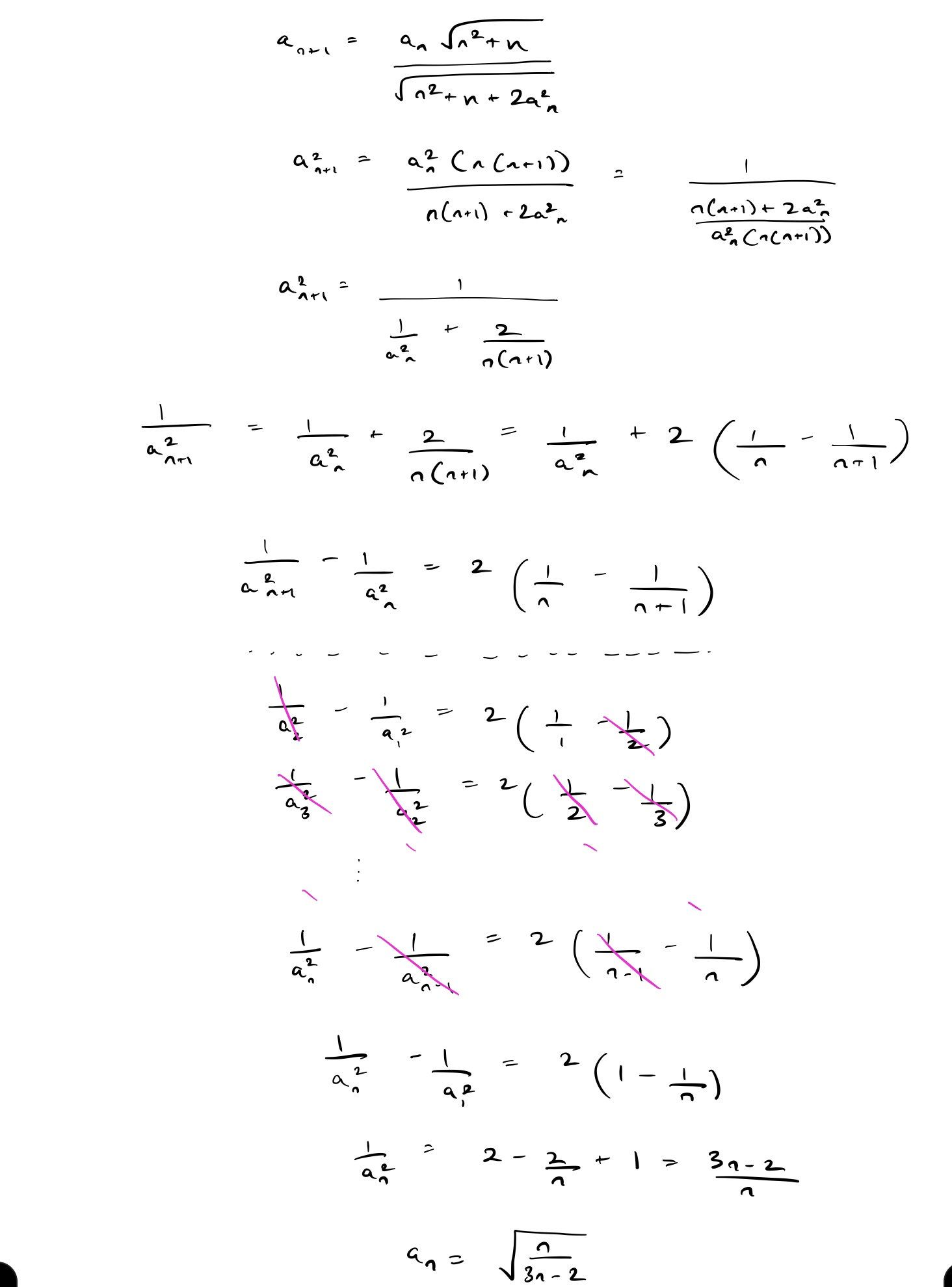# Help: Sequence

A sequence {$a_{n}$} of real numbers is defined by $a_{1}=1$ and for all integers $n≥1$

$a_{n+1}=\frac{a_{n}\sqrt{n²+n}}{\sqrt{n²+n+2a_{n}²}}$

Compute the sum of all positive integers $n<1000$ for which $a_{n}$ is a rational number.Note by Ryan Merino
5 months ago

This discussion board is a place to discuss our Daily Challenges and the math and science related to those challenges. Explanations are more than just a solution — they should explain the steps and thinking strategies that you used to obtain the solution. Comments should further the discussion of math and science.

When posting on Brilliant:

• Use the emojis to react to an explanation, whether you're congratulating a job well done , or just really confused .
• Ask specific questions about the challenge or the steps in somebody's explanation. Well-posed questions can add a lot to the discussion, but posting "I don't understand!" doesn't help anyone.
• Try to contribute something new to the discussion, whether it is an extension, generalization or other idea related to the challenge.

MarkdownAppears as
*italics* or _italics_ italics
**bold** or __bold__ bold
- bulleted- list
• bulleted
• list
1. numbered2. list
1. numbered
2. list
Note: you must add a full line of space before and after lists for them to show up correctly
paragraph 1paragraph 2

paragraph 1

paragraph 2

[example link](https://brilliant.org)example link
> This is a quote
This is a quote
    # I indented these lines
# 4 spaces, and now they show
# up as a code block.

print "hello world"
# I indented these lines
# 4 spaces, and now they show
# up as a code block.

print "hello world"
MathAppears as
Remember to wrap math in $$ ... $$ or $ ... $ to ensure proper formatting.
2 \times 3 $2 \times 3$
2^{34} $2^{34}$
a_{i-1} $a_{i-1}$
\frac{2}{3} $\frac{2}{3}$
\sqrt{2} $\sqrt{2}$
\sum_{i=1}^3 $\sum_{i=1}^3$
\sin \theta $\sin \theta$
\boxed{123} $\boxed{123}$

Sort by:- 5 months ago

Hopefully you can continue from here( I have no clue on how to find out when $a_n$ is rational, or maybe I haven’t put enough thought on that part)

- 5 months ago

Thanks for this big help mate, just need to compute when the numerator, $n$, is a perfect and at the same time the denominator, $3n-2$, is also a perfect square so that $a_{n}$ is a rational number. Found out that only when $n=1, 9$, and $121$ works, so the sum is $131$. I don't have a clue for when $3n-2$ is a perfect square when $n$ is a perfect square so I just tried all perfect squares less than $1000$.

- 5 months ago

There can be times where common factors cancel out to give a square

- 5 months ago

Thank you for the correction mate. How careless of me, now I have a headache answering this. lol

- 5 months ago

I did some simplifications and checked using a program all the 1000 possibilities and tother that the ones mentioned none of the others looked rational(I had to manually check all, so I could have made a mistake)

- 5 months ago

Still it was wrong of me to presume that $a_{n}$ will only be rational $iff$ $n$ and $3n-2$ are perfect squares. Thanks for all the help mate, really appreciated. :)

- 5 months ago

No problem at all

- 5 months ago

If you do assume both have to be squares though,a little modular arithmetic taken against four, will lead to the conclusion that $n$ is of the form $4k+1$

- 5 months ago

I recently thought of this back again and found out that your hypothesis that both numerator and denominator are both squares is indeed true, the only factor $n$ and $3n-2$ can share is two, putting $n=k^2,2k^2$ and $3n-2=m^2,2m^2$ for the two conditions where they share no factor and share a two, the equation becomes

$3k^2-2=m^2$ And $3k^2-1=m^2$

Doing modular arithmetic with respect to three on both sides knowing that $m^2 \mod 3 = 0,1$, it’s easy to show that the second doesn’t satisfy ( it gives $2 \mod 3$ on LHS) and therefore only the case where the numbers share no common factors can be taken

- 4 months, 3 weeks ago

@Jason Gomez Thank you mate, now it's all clear to me. :)

- 4 months, 3 weeks ago